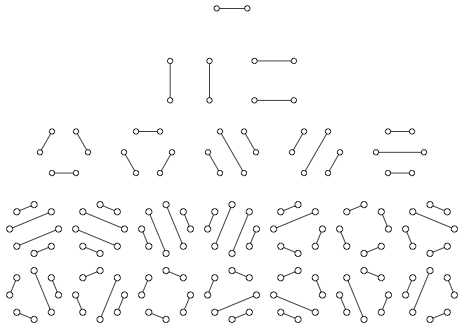# Glossary

## Catalan numbers

The Catalan number $c_n$ counts the total number of noncrossing perfect matchings in the complete graph $K_{2n}$. We can see that $c_1 = 1$, and we set $c_0 = 1$ as well. For a general $n$, say that the nodes of $K_{2n}$ are labeled with the positive integers from 1 to $n$ and ordered around a circle. We can join node 1 to any of the remaining $2n - 1$ nodes; yet once we have chosen this node (say $m$), we cannot add another edge to the matching that crosses the edge $\{1, m\}$. As a result, we must match all the edges on one side of $\{1, m\}$ to each other. This requirement forces $m$ to be even, so that we can write $m = 2k$ for some positive integer $k$.

There are $2k - 2$ nodes on one side of $\{1, m\}$ and $2n - 2k$ nodes on the other side of $\{1, m\}$, so that in turn there will be $c_{k-1} \cdot c_{n - k}$ different ways of forming a perfect matching on the remaining nodes of $K_{2n}$. If we let $m$ vary over all possible $n - 1$ choices of even numbers between 1 and $2n$, then we obtain the recurrence relation $c_n = \sum_{k = 1}^{n}{c_{k-1} \cdot c_{n-k}}$, which helps us count the Catalan numbers via dynamic programming.

The first four Catalan numbers (1, 2, 5, and 14) are counted by the figure below, which shows all possible noncrossing perfect matchings of complete graphs on 2, 4, 6, and 8 nodes.